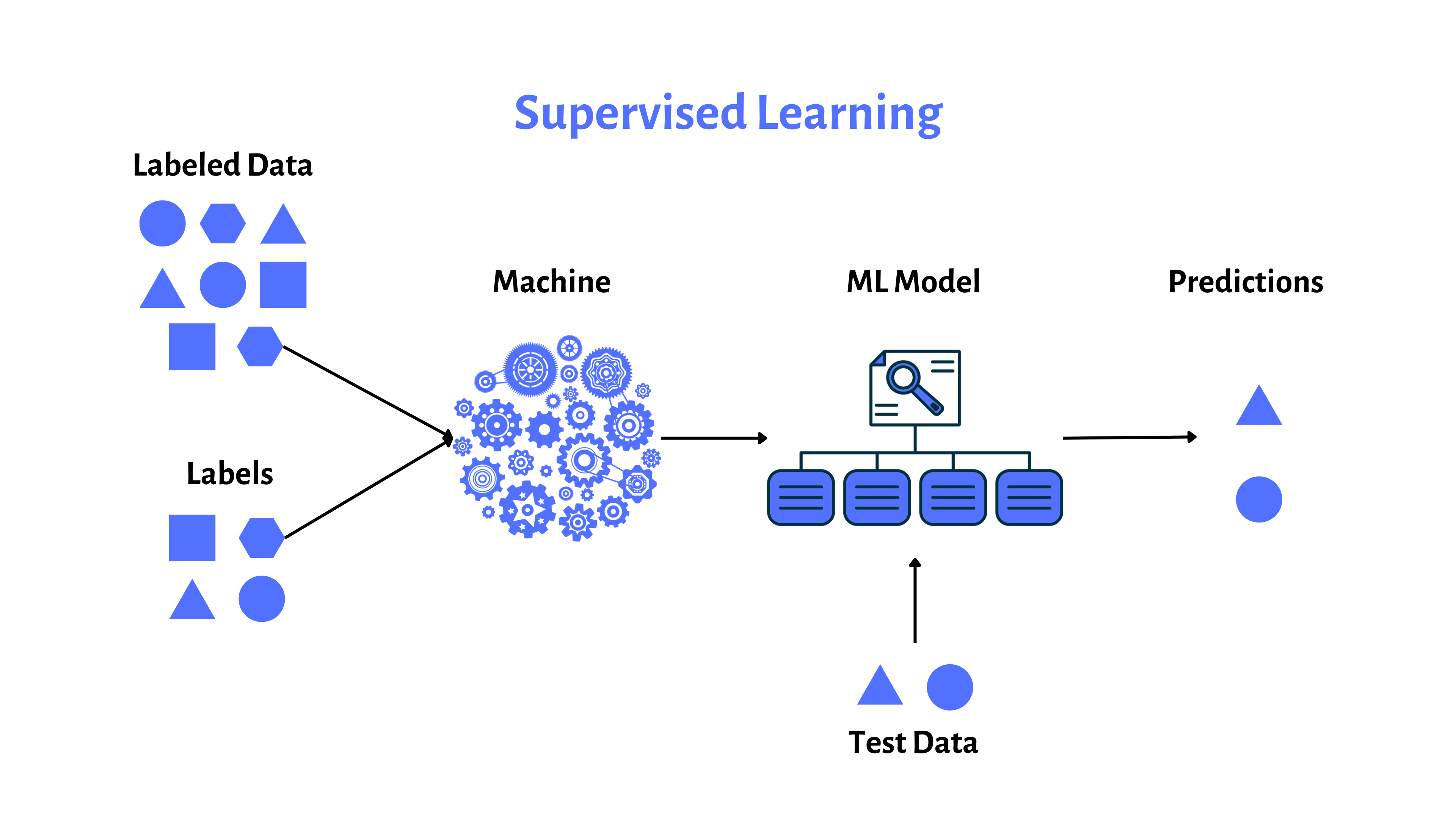# Supervised Learning

Supervised learning is the most common form of machine learning, where an algorithm is trained using a labeled dataset. The dataset contains input-output pairs, with the output (label) being the correct answer for each input. The algorithm learns the relationship between inputs and outputs and can then make predictions on unseen data. Examples of supervised learning tasks include classification (categorizing data into classes) and regression (predicting a continuous value).